# Bonbons 2

Kilo sweets will cost 260 CZK. The first type has a price per 320 kg, the second type 240 CZK per kg. How many kilos of both kinds of sweets need to prepare a 100 kg mixture ?

Result

x =  25 kg
y =  75 kg

#### Solution:

320x + 240y = 260(x+y)
x+y=100

60x-20y = 0
x+y = 100

x = 25
y = 75

Calculated by our linear equations calculator.

Leave us a comment of example and its solution (i.e. if it is still somewhat unclear...):

Showing 0 comments:Be the first to comment!#### To solve this verbal math problem are needed these knowledge from mathematics:

Do you have a system of equations and looking for calculator system of linear equations?

## Next similar examples:

1. Coffee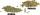Coffee merchant has coffee robusta and arabica species. 1 kg Robusta worth 450 CZK, Arabica 1 kg is 300 CZK more expensive. Calculate how many kilograms of Robusta and Arabica will need to produce 30 kg of the mixture so that the mixture cost is 490 CZK p
2. MixingIf we mix 5 kg of goods of one kind and 3 kg second one, resulting mixture cost 16.50 EUR/kg. If these quantities are mixed in reverse - first three kilograms and 5 kilograms second cost of mixture is 18.50 EUR/kg. What is the price of one kg of goods of.
3. CoffeeIn stock are three kinds of branded coffee prices: I. kind......248 Kč/kg II. kind......134 Kč/kg III. kind.....270 Kč/kg Mixing these three species in the ratio 10:7:7 create a mixture. What will be the price of 1100 grams of this mixture?
4. Tea blendTea blends are maked from two kinds of tea. In standard tea mixture are two teas in the ratio 1:3 and 40 g costs 42 CZK. In the premium tea mixture are weighing two teas in the ratio 1:1 and 50 grams costs 60 CZK. How much cost 10 grams of more expensive k
5. Two wine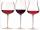In 2:1 mix wine solution cost 4.1 USD. In 1:2 mix wine solution cost 4.5 USD. How much cost liter of each wine?
6. Alloy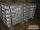The first alloy is a mixture of two metals in the ratio 1:2, the second is a mixture of same metals in the ratio 2:3. At what ratio we have these two alloys put into the furnace to obtain a new metal alloy with ratio 17:27? (All three ratios correspond to.
7. Alcohol 2Two types of alcohol one 63% and second 75% give 20 liters of 69% alcohol. How many liters of each type are in the mixture?
8. Ladislav in Amsterdam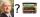Ladislav was reward and went to conference in Amsterdam. Conferense fee was € 3484. Calculate how many books Ladislav could buy at the price of 48 and 52 euros if he don't want fill whole home library and he can buy only 70 books?
9. PharmacyAt the pharmacy are in one container 20% solution in the second 50% solution of disinfectant. They need to prepare 4 L of 48-percent solution. What amount of solution from each container is needed to mix?
10. Metal alloy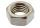What is the ratio of metals in the alloy that is in the 50 tonnes of steel to 30 kg nickel?
11. Crown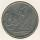Siblings collect a 2-crowns and 5-crowns. Together have 80 coins with a total of value 310 crowns. How many saved two-crowns and how many five-crowns?
12. SpiritFrom 55% and 80% spirit we would like to produce 0.2 kg of 60% spirit. How many of them we must use in a solution?
13. Solution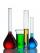In 469 dl red solution is 84 dl red color and in 102 dl blue solution is 52 dl blue color. How many dl of red and blue dl color solution must be mixed to get a mixture of 247 dl contain 116 dl of color?
14. Water reservoir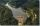The water reservoir is filled through one inlet 4 hours later than both together, then another inlet 9 hours later than both together. For how long is filled by each separately?
15. Cottages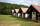The summer camp is 41 cottages. Rooms are for three and for four in them. How many of the 140 campers lives of three?
16. SolutionWhat amount of 80% solution and what amount of water is needed to mix in order to obtain two liters of 60% solution of this mixture?
17. RingThe ring made from gold and copper alloy has a weight of 14.5 g and a volume of 1.03 cm3. How much gold and how much copper it contains? The metal densities are Au 19.3 g/cm³ and Cu 8.94 kg·dm-3